# Vue 响应式原理模拟

## 一、三个概念

### 1. 数据驱动

• 数据响应式
• 数据模型仅仅是普通的 JavaScript 对象，而当我们修改数据时，视图会进行更新，避免了繁琐的 DOM 操作，提高开发效率
• 双向绑定
• 数据改变，视图改变；视图改变，数据也随之改变
• 我们可以使用 v-model 在表单元素上创建双向数据绑定
• 数据驱动是 Vue 最独特的特性之一
• 开发过程中仅需要关注数据本身，不需要关心数据是如何渲染到视图

### 2. 数据响应式核心原理

• Vue 2.x
// 模拟 Vue 中的 data 选项
let data = {
msg: 'hello'
}
// 模拟 Vue 的实例
let vm = {}
// 数据劫持：当访问或者设置 vm 中的成员的时候，做一些干预操作
Object.defineProperty(vm, 'msg', {
// 可枚举（可遍历）
enumerable: true,
// 可配置（可以使用 delete 删除，可以通过 defineProperty 重新定义）
configurable: true,
// 当获取值的时候执行
get () {
console.log('get: ', data.msg)
return data.msg
},
// 当设置值的时候执行
set (newValue) {
console.log('set: ', newValue)
if (newValue === data.msg) {
return
}
data.msg = newValue
// 数据更改，更新 DOM 的值
document.querySelector('#app').textContent = data.msg
}
})
// 测试
vm.msg = 'Hello World'
console.log(vm.msg)

• Vue 3.x
// 模拟 Vue 中的 data 选项
let data = {
msg: 'hello',
count: 0
}
// 模拟 Vue 实例
let vm = new Proxy(data, {
// 当访问 vm 的成员会执行
get (target, key) {
console.log('get, key: ', key, target[key])
return target[key]
},
// 当设置 vm 的成员会执行
set (target, key, newValue) {
console.log('set, key: ', key, newValue)
if (target[key] === newValue) {
return
}
target[key] = newValue
document.querySelector('#app').textContent = target[key]
}
})
// 测试
vm.msg = 'Hello World'
console.log(vm.msg)

### 3. 发布订阅模式和观察者模式

#### Ⅰ. 发布订阅模式

• 发布/订阅模式
• 订阅者
• 发布者
• 信号中心

• Vue 的自定义事件
let vm = new Vue()
vm.$on('dataChange', () => { console.log('dataChange') }) vm.$on('dataChange', () => {
console.log('dataChange1')
})
vm.$emit('dataChange') 复制代码 • 兄弟组件通信过程 // eventBus.js // 事件中心 let eventHub = new Vue() // ComponentA.vue // 发布者 addTodo: function () { // 发布消息(事件) eventHub.$emit('add-todo', { text: this.newTodoText })
this.newTodoText = ''
}
// ComponentB.vue
// 订阅者
created: function () {
// 订阅消息(事件)
eventHub.$on('add-todo', this.addTodo) } 复制代码 • 模拟 Vue 自定义事件的实现 class EventEmitter { constructor () { // { eventType: [ handler1, handler2 ] } this.subs = {} } // 订阅通知$on (eventType, handler) {
this.subs[eventType] = this.subs[eventType] || []
this.subs[eventType].push(handler)
}
// 发布通知
$emit (eventType) { if (this.subs[eventType]) { this.subs[eventType].forEach(handler => { handler() }) } } } // 测试 var bus = new EventEmitter() // 注册事件 bus.$on('click', function () {
console.log('click')
})
bus.$on('click', function () { console.log('click1') }) // 触发事件 bus.$emit('click')

#### Ⅱ. 观察者模式

• 观察者(订阅者) -- Watcher
• update()：当事件发生时，具体要做的事情
• 目标(发布者) -- Dep
• subs 数组：存储所有的观察者
• notify()：当事件发生，调用所有观察者的 update() 方法
• 没有事件中心
// 目标(发布者)
// Dependency
class Dep {
constructor () {
// 存储所有的观察者
this.subs = []
}
// 添加观察者
if (sub && sub.update) {
this.subs.push(sub)
}
}
// 通知所有观察者
notify () {
this.subs.forEach(sub => {
sub.update()
})
}
}
// 观察者(订阅者)
class Watcher {
update () {
console.log('update')
}
}
// 测试
let dep = new Dep()
let watcher = new Watcher()
dep.notify()

#### Ⅲ. 总结

• 观察者模式是由具体目标调度，比如当事件触发，Dep 就会去调用观察者的方法，所以观察者模式的订阅者与发布者之间是存在依赖的。
• 发布/订阅模式由统一调度中心调用，因此发布者和订阅者不需要知道对方的存在。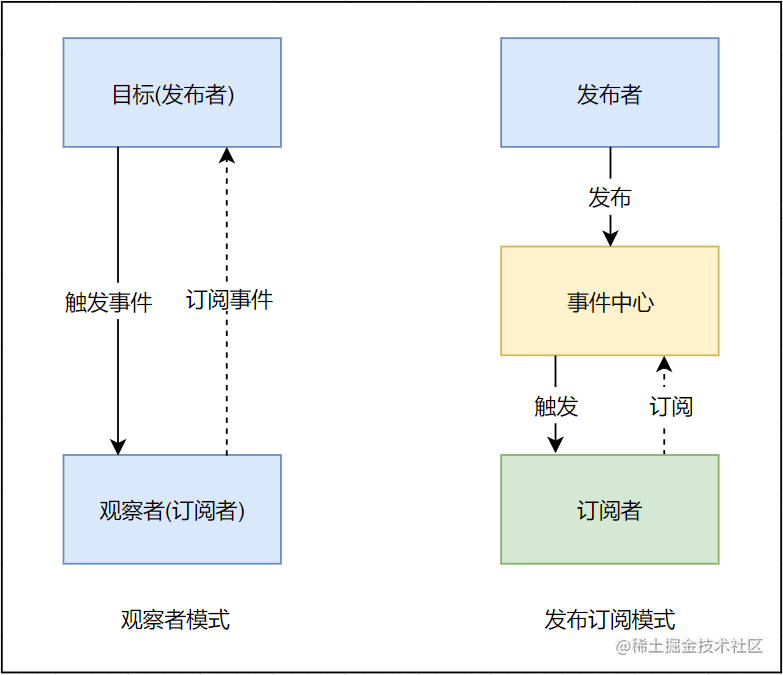## 二、Vue 响应式原理模拟

### 1. Vue

• 功能
• 负责接收初始化的参数(选项)
• 负责把 data 中的属性注入到 Vue 实例，转换成 getter/setter
• 负责调用 observer 监听 data 中所有属性的变化
• 负责调用 compiler 解析指令/插值表达式
• 结构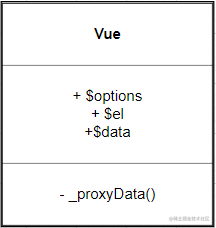• 实现
class Vue {
constructor (options) {
// 1. 保存选项的数据
this.$options = options || {} this.$data = options.data || {}
const el = options.el
this.$el = typeof options.el === 'string' ? document.querySelector(el) : el // 2. 负责把 data 注入到 Vue 实例 this._proxyData(this.$data)
// 3. 负责调用 Observer 实现数据劫持
new Observer(this.$data) // 4. 负责调用 Compiler 解析指令/插值表达式等 new Compiler(this) } _proxyData (data) { // 遍历 data 的所有属性 Object.keys(data).forEach(key => { Object.defineProperty(this, key, { get () { return data[key] }, set (newValue) { if (data[key] === newValue) { return } data[key] = newValue } }) }) } } 复制代码 ### 2. Observer • 功能 • 负责把 data 选项中的属性转换成响应式数据 • data 中的某个属性也是对象，把该属性转换成响应式数据 • 数据变化发送通知 • 结构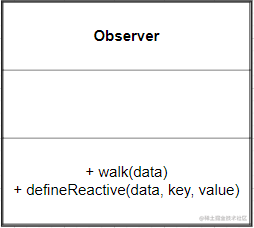• 实现 // 负责数据劫持 // 把$data 中的成员转换成 getter/setter
class Observer {
constructor(data) {
this.walk(data)
}
// 1. 判断数据是否是对象，如果不是对象返回
// 2. 如果是对象，遍历对象的所有属性，设置为 getter/setter
walk(data) {
if (!data || typeof data !== 'object') {
return
}
// 遍历 data 的所有成员
Object.keys(data).forEach(key => {
this.defineReactive(data, key, data[key])
})
}
// 定义响应式成员
defineReactive(data, key, val) {
const that = this
// 如果 val 是对象，继续设置它下面的成员为响应式数据
this.walk(val)
Object.defineProperty(data, key, {
configurable: true,
enumerable: true,
get() {
return val
},
set(newValue) {
if (newValue === val) {
return
}
// 如果 newValue 是对象，设置 newValue 的成员为响应式
that.walk(newValue)
val = newValue
}
})
}
}

### 3. Compiler

• 功能
• 负责编译模板，解析指令/插值表达式
• 负责页面的首次渲染
• 当数据变化后重新渲染视图
• 结构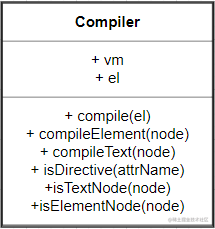• 实现

① compile()

// 负责解析指令/插值表达式
class Compiler {
constructor(vm) {
this.vm = vm
this.el = vm.$el // 编译模板 this.compile(this.el) } // 编译模板 // 处理文本节点和元素节点 compile(el) { const nodes = el.childNodes Array.from(nodes).forEach(node => { // 判断是文本节点还是元素节点 if (this.isTextNode(node)) { this.compileText(node) } else if (this.isElementNode(node)) { this.compileElement(node) } if (node.childNodes && node.childNodes.length) { // 如果当前节点中还有子节点，递归编译 this.compile(node) } }) } // 判断是否是文本节点 isTextNode(node) { return node.nodeType === 3 } // 判断是否是属性节点 isElementNode(node) { return node.nodeType === 1 } // 判断是否是以 v- 开头的指令 isDirective(attrName) { return attrName.startsWith('v-') } // 编译文本节点 compileText(node) { } // 编译属性节点 compileElement(node) { } } 复制代码 ② compileText() // 编译文本节点 compileText(node) { const reg = /\{\{(.+)\}\}/ // 获取文本节点的内容 const value = node.textContent if (reg.test(value)) { // 插值表达式中的值就是我们要的属性名称 const key = RegExp.$1.trim()
// 把插值表达式替换成具体的值
node.textContent = value.replace(reg, this.vm[key])
}
}

③ compileElement()

• 负责编译元素的指令
• 处理 v-text 的首次渲染
• 处理 v-model 的首次渲染
// 编译属性节点
compileElement(node) {
// 遍历元素节点中的所有属性，找到指令
Array.from(node.attributes).forEach(attr => {
// 获取元素属性的名称
let attrName = attr.name
// 判断当前的属性名称是否是指令
if (this.isDirective(attrName)) {
// attrName 的形式 v-text v-model
// 截取属性的名称，获取 text model
attrName = attrName.substr(2)
// 获取属性的名称，属性的名称就是我们数据对象的属性 v-text="name"，获取的是name
const key = attr.value
// 处理不同的指令
this.update(node, key, attrName)
}
})
}
// 负责更新 DOM
// 创建 Watcher
update(node, key, dir) {
// node 节点，key 数据的属性名称，dir 指令的后半部分
const updaterFn = this[dir + 'Updater']
updaterFn && updaterFn(node, this.vm[key])
}
// v-text 指令的更新方法
textUpdater(node, value) {
node.textContent = value
}
// v-model 指令的更新方法
modelUpdater(node, value) {
node.value = value
}

### 4. Dep

• 功能
• 收集依赖，添加观察者(watcher)
• 通知所有观察者
• 结构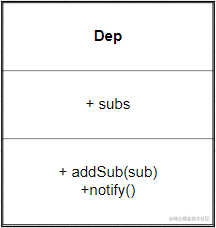• 实现
class Dep {
constructor() {
// 存储所有的观察者
this.subs = []
}
// 添加观察者
if (sub && sub.update) {
this.subs.push(sub)
}
}
// 通知所有观察者
notify() {
this.subs.forEach(sub => {
sub.update()
})
}
}
// 以下代码在 Observer 类中 defineReactive 方法中添加
// 创建 dep 对象收集依赖
const dep = new Dep()
// getter 中
// get 的过程中收集依赖
// setter 中
// 当数据变化之后，发送通知
dep.notify()

### 5. Watcher

• 功能
• 当数据变化触发依赖， dep 通知所有的 Watcher 实例更新视图
• 自身实例化的时候往 dep 对象中添加自己
• 结构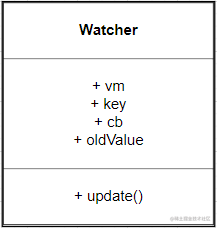• 实现
class Watcher {
constructor(vm, key, cb) {
this.vm = vm
// data 中的属性名称
this.key = key
// 当数据变化的时候，调用 cb 更新视图
this.cb = cb
// 在 Dep 的静态属性上记录当前 watcher 对象，当访问数据的时候把 watcher 添加到dep 的 subs 中
Dep.target = this
// 触发一次 getter，让 dep 为当前 key 记录 watcher
this.oldValue = vm[key]
// 清空 target
Dep.target = null
}
update() {
const newValue = this.vm[this.key]
if (this.oldValue === newValue) {
return
}
this.cb(newValue)
}
}

// 在 compiler.js(即Compiler类) 中为每一个指令/插值表达式创建 watcher 对象，监视数据的变化
compileText(node) {
const reg = /\{\{(.+?)\}\}/
const value = node.textContent
if (reg.test(value)) {
const key = RegExp.\$1.trim()
node.textContent = value.replace(reg, this.vm[key])
// 编译差值表达式中创建一个 watcher，观察数据的变化
new Watcher(this.vm, key, newValue => {
node.textContent = newValue
})
}
}
// 因为在 textUpdater等中要使用 this
updaterFn && updaterFn.call(this, node, this.vm[key], key)
// v-text 指令的更新方法
textUpdater(node, value, key) {
node.textContent = value
// 每一个指令中创建一个 watcher，观察数据的变化
new Watcher(this.vm, key, value => {
node.textContent = value
})
}

// 视图变化更新数据
// v-model 指令的更新方法
modelUpdater(node, value, key) {
node.value = value
// 每一个指令中创建一个 watcher，观察数据的变化
new Watcher(this.vm, key, value => {
node.value = value
})
// 监听视图的变化
this.vm[key] = node.value
})
}

## 三、总结

### 1. 两个问题你会了吗

• 给属性重新赋值成对象，是否是响应式的？
• 给 Vue 实例新增一个成员是否是响应式的？

### 2. 通过下图回顾整体流程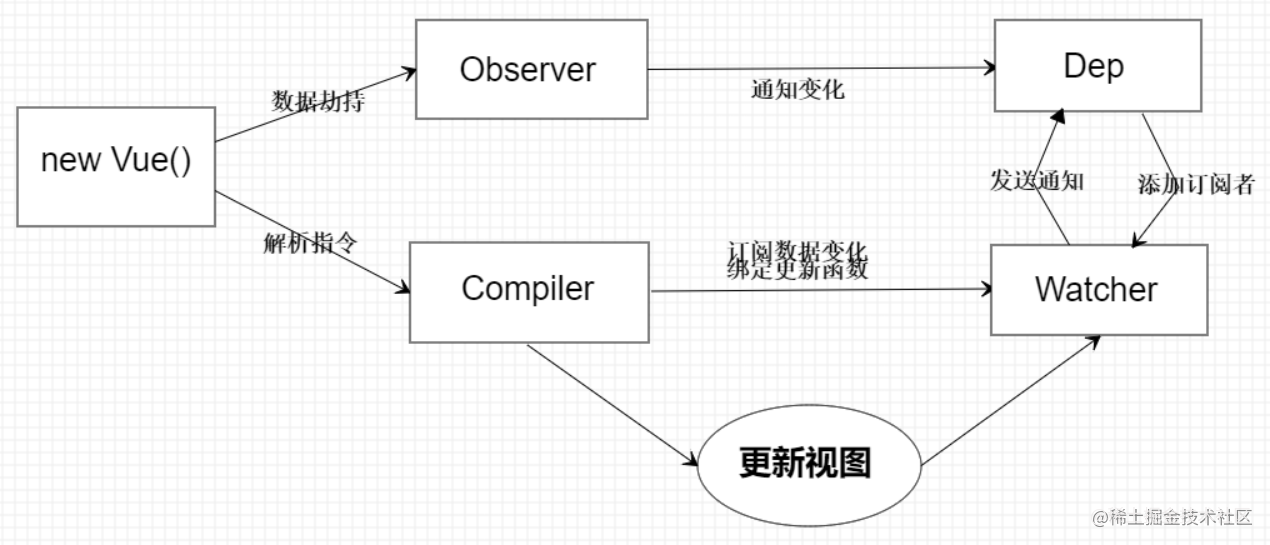### 3. Vue

• 记录传入的选项，设置 $data/$el
• 把 data 的成员注入到 Vue 实例
• 负责调用 Observer 实现数据响应式处理（数据劫持）
• 负责调用 Compiler 编译指令/插值表达式等

### 4. Observer

• 数据劫持
• 负责把 data 中的成员转换成 getter/setter
• 负责把多层属性转换成 getter/setter
• 如果给属性赋值为新对象，把新对象的成员设置为 getter/setter
• 添加 Dep 和 Watcher 的依赖关系
• 数据变化发送通知

### 5. Compiler

• 负责编译模板，解析指令/插值表达式
• 负责页面的首次渲染过程
• 当数据变化后重新渲染

### 6. Dep

• 收集依赖，添加订阅者(watcher)
• 通知所有订阅者

### 7. Watcher

• 自身实例化的时候往dep对象中添加自己
• 当数据变化dep通知所有的 Watcher 实例更新视图